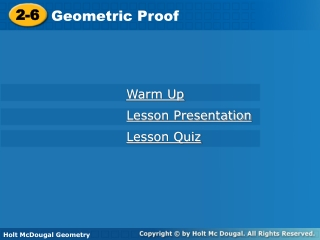DownloadDownload Presentation2-6

# 2-6

Télécharger la présentation## 2-6

- - - - - - - - - - - - - - - - - - - - - - - - - - - E N D - - - - - - - - - - - - - - - - - - - - - - - - - - -
##### Presentation Transcript

1. 2-6 Geometric Proof Warm Up Lesson Presentation Lesson Quiz Holt McDougal Geometry Holt Geometry

2. Warm Up Determine whether each statement is true or false. If false, give a counterexample. 1.It two angles are complementary, then they are not congruent. 2. If two angles are congruent to the same angle, then they are congruent to each other. 3. Supplementary angles are congruent. false; 45° and 45° true false; 60° and 120°

3. Objectives Write two-column proofs. Prove geometric theorems by using deductive reasoning.

4. Vocabulary theorem two-column proof

5. Definitions • Postulates • Properties • Theorems Conclusion Hypothesis When writing a proof, it is important to justify each logical step with a reason. You can use symbols and abbreviations, but they must be clear enough so that anyone who reads your proof will understand them.

6. Example 1: Writing Justifications Write a justification for each step, given that A and Bare supplementary and mA = 45°. 1. Aand Bare supplementary. mA = 45° Given information Def. of supp s 2. mA+ mB= 180° Subst. Prop of = 3. 45°+ mB= 180° Steps 1, 2 Subtr. Prop of = 4. mB= 135°

7. Helpful Hint When a justification is based on more than the previous step, you can note this after the reason, as in Example 1 Step 3.

8. Write a justification for each step, given that B is the midpoint of AC and ABEF. 1. Bis the midpoint of AC. 2. AB BC 3. AB EF 4. BC EF Check It Out! Example 1 Given information Def. of mdpt. Given information Trans. Prop. of 

9. A theorem is any statement that you can prove. Once you have proven a theorem, you can use it as a reason in later proofs.

10. A geometric proof begins with Given and Prove statements, which restate the hypothesis and conclusion of the conjecture. In a two-column proof, you list the steps of the proof in the left column. You write the matching reason for each step in the right column.

11. Example 2: Completing a Two-Column Proof Fill in the blanks to complete the two-column proof. Given: XY Prove: XY  XY Reflex. Prop. of =

12. Check It Out! Example 2 Fill in the blanks to complete a two-column proof of one case of the Congruent Supplements Theorem. Given: 1 and 2 are supplementary, and 2 and 3 are supplementary. Prove: 1  3 Proof: • 1 and 2 are supp., and 2 and 3 are supp. b. m1 + m2 = m2 + m3 c. Subtr. Prop. of = d. 1  3

13. Before you start writing a proof, you should plan out your logic. Sometimes you will be given a plan for a more challenging proof. This plan will detail the major steps of the proof for you.

14. Helpful Hint If a diagram for a proof is not provided, draw your own and mark the given information on it. But do not mark the information in the Prove statement on it.

15. Example 3: Writing a Two-Column Proof from a Plan Use the given plan to write a two-column proof. Given: 1 and 2 are supplementary, and 1  3 Prove: 3 and 2 are supplementary. Plan: Use the definitions of supplementary and congruent angles and substitution to show that m3 + m2 = 180°.By the definition of supplementary angles, 3 and 2are supplementary.

16. Example 3 Continued Given 1 and 2 are supplementary. 1  3 m1+ m2 = 180° Def. of supp. s m1= m3 Def. of s Subst. m3+ m2 = 180° Def. of supp. s 3 and 2 are supplementary

17. Check It Out! Example 3 Use the given plan to write a two-column proof if one case of Congruent Complements Theorem. Given: 1 and 2 are complementary, and 2 and 3 are complementary. Prove: 1  3 Plan: The measures of complementary angles add to 90° by definition. Use substitution to show that the sums of both pairs are equal. Use the Subtraction Property and the definition of congruent angles to conclude that 1 3.

18. Check It Out! Example 3 Continued Given 1 and 2 are complementary. 2 and 3 are complementary. m1+ m2 = 90° m2+ m3 = 90° Def. of comp. s m1+ m2 = m2+ m3 Subst. Reflex. Prop. of = m2= m2 m1 = m3 Subtr. Prop. of = 1  3 Def. of  s

19. Lesson Quiz: Part I Write a justification for each step, given that mABC= 90° and m1= 4m2. 1. mABC= 90° and m1= 4m2 2. m1+ m2 = mABC 3. 4m2 + m2 = 90° 4. 5m2= 90° 5. m2= 18° Given  Add. Post. Subst. Simplify Div. Prop. of =.

20. Lesson Quiz: Part II 2. Use the given plan to write a two-column proof. Given: 1, 2 , 3, 4 Prove: m1 + m2 = m1 + m4 Plan: Use the linear Pair Theorem to show that the angle pairs are supplementary. Then use the definition of supplementary and substitution. 1. 1 and 2 are supp. 1 and 4 are supp. 1. Linear Pair Thm. 2. Def. of supp. s 2. m1+ m2 = 180°, m1+ m4 = 180° 3. Subst. 3. m1+ m2 = m1+ m4